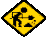# Traveling Salesman Problem (TSP)

2009 Jan 16### Definition and Discussion

The mathematical statement of the problem is two fold: minimize the total travel cost function, and visit each node of the graph exactly once. In real world problems the second requirement would be restated as "visit each node at least once". This could allow a possible, and more optimal, smaller total travel cost. Once the requirement of visting each node is dropped in favor of visting each node at least once, it also becomes possible to drop the requirement to end where you started. This makes subsequent solutions have different starting states.

If it is given that the graph has all n nodes connected (i.e. there are not disjoint unconneced sub-graphs) and a path exists to any node from every other node, in the worst case by naive brute force (since the starting point does not matter), there are (n-1)! cases to examine.

The actual graph could be fully connected or not. If not fully connect it may not be possible to make a circuit, as is well known. The simplest is because the graph is a tree and has no loops - a circuit (or closed path) is not possible, or in more complex cases because it is impossible by known graph theorems.

The cost function in a simple case could be the number of hops, i.e. each arc between a pair of connected nodes has unit cost. Another cost could be the geometric distance between nodes in d dimensions. More complex cost functions could have multiple factors: time, distance, money (where time and money may not be independent), quality of travel, requirement for air conditioning, sleep over delays, and so on.

Note that for the one dimensional problem this algorithm has no circuit, every node is connect to at most two neighbors, and the algorithm is identical to sorting. TSP is only challenging in 2 or more dimensions.

Real world factors or constraints include many possibilities

• The graph is not fully connected, there may not be a path between every pair of nodes.
• The cost is different in different directions.
• Travel from node a to b may be possible but the symmetric travel from node b to a is not possible.
• There may be multiple paths between two given nodes with different cost.
• The time that travel between nodes can actually take place for any particular path is constrained (e.g. in bus, train, boat, and air travel).
• Upper bound limit on total travel time or money cost.
• Absolute bound on total travel time or travel time between a particular pair of nodes.

There are further non linear constarints in scheduled travel where there must be a minimum delay between arrival at a node and departure from the node for: unloading time, transportation time within the node and loading time. For a person this can vary depending on whether there is carry-on baggage or checked baggage. For material transport there may be time needed to record receipt and transhipment of each item, or storage insertio and later retreival of an item not being shipped out in the near future. Such problems may need an event driven simulator which models these queueing aspects.

### Exact and Near Optimal Solutions

The brute force exact solution of (n-1)! cases to examine is, for not very large n, impractical in terms of computer time and memory. Typical memories are measured in GB (230==1073741824), which compares with only (13!==6227020800). Assume it takes 100ns (nanoseconds) to examine each case, meaning there can be ten million, 107, cases examined per second. Then if an answer is wanted in a reasonable time, say one week which is (7*24*60*60==604800) seconds, then the total time limit is: 6.048*1012 cases examined in a week which compares with only (15!=1307674368000).

One sub-optimal solution uses the method where some nodes are clustered in a small number of groups, each with a hub. The nodes in the cluster only allow travel between themself and the hub node - direct travel between nodes in a group excluding the group hub node is not possible. Then a second level is added where all hubs are fully (or partially) connected. Another variation is to have a master hub which only allows travel between itself and the other group hubs.

There are sub-optimal algorithms which do very well in time to find a solution and give acceptable total cost. These algorithms include variations of so called greedy methods where an arc between two nodes is taken between one node and its closest neighbor. For such greedy algorithms, a first step is sometimes to sort the distance between allowed node travel in increasing distance before clustering. Then create clusters starting with the smallest distances between node pairs. The variations decide how to group and modify these clusters of connected nodes when the clusters merge. In more comple algorithms it is necessary to remember and use the conditions (e.g. distance cost and nodes) when clusters merge.